# ICvectorfields: Producing vector fields from spatial time series data

library(ICvectorfields)
library(ggplot2)
library(ggnewscale)
library(metR)
library(terra)
#> terra version 1.3.22
library(ncf)

# Overview

The package name ICvectorfields stands for Image Correlation vector fields. The ICvectorfields package is designed to facilitate vector field construction and visualization using empirical data. Vector fields are heavily used in mathematics and meteorology to represent system dynamics. They comprise multiple spatially separated vectors or arrows that point in the direction of movement and whose length represents displacement magnitude. In many systems movement is not unidirectional nor is movement speed spatially constant. Picture a meteorological wind field for example: Wind speeds and directions are complex functions of underlying topography and atmospheric dynamics. As a result, wind fields are quite variable when visualized at a regional scale. In such systems a single wind vector or speed metric does not describe spatiotemporal patterns in the data well and movement in the system is better visualized using a vector field. The techniques used to construct vector fields in ICvectorfields are inspired by the the digital image correlation technique that is often abbreviated to DIC. However, an extension of the DIC technique has been implemented in ICvectorfields, which is designed to quantify persistent movement in spatial time series data that extend beyond two time instances.

## Digital Image Correlation approach

A detailed mathematical and algorithmic description of the approach used in ICvectorfields will be presented elsewhere. Here a brief summary of the general DIC technique is presented. When first conceived, DIC was nothing more than a comparison of two digital images using two-dimensional cross-correlation or cross-covariance (Anuta 1970). Currently, the digital image correlation approach has been widely adopted in engineering and materials science as a method for estimating displacement, strain, and torsion when a force is applied to a planar material (Sutton, Orteu, and Schreier 2009). Specifically, the surface of the material of interest is speckled and then photographed multiple times before, during, and after force application. Comparison of sequences of images using digital image correlation allows calculation of displacement based on movement of speckles from one image to the next. More complex calculations produce estimates of strain and torsion. In modern implementations of DIC, regions of interest can be defined before analysis and displacement is estimated based on where cross-covariance between series of images is maximized.

## 2D Cross-covariance in the ICvectorfields package

The most efficient algorithms for two-dimensional cross-correlation exploit discrete fast Fourier transforms (Anuta 1970) and return a two-dimensional surface of cross-correlation or cross-covariance estimates as does the Xcov2D function in ICvectorfields. Along with typical displacement estimation using the DIC technique, ICvectorfields also includes a novel algorithm for estimating persistent movement direction and speed. Because it is quantifying persistent movement, the novel algorithm requires a stack of at least three images in chronological sequence. The stack of images is a three-dimensional array with two planar space dimensions and a time dimension. The three-dimensional array is replicated and then lagged by removing a user-specified number of layers from the top of one 3D-array and the bottom of the other. Then, data in focal regions and comparison regions are dimension reduced by averaging either along rows or columns in the pixelated images. Two dimensional cross-covariance is computed using four pairs of images that have one space dimension and one time dimension. One pair of images is the lagged and unlagged spatiotemporal matrices that have been row-averaged and the other is the lagged and unlagged spatiotemporal matrices that have been column-averaged. Orthogonal velocity vectors in the vertical and horizontal directions are computed using two-dimensional cross-covariance. In the remainder of this document, the novel algorithm will be called Space Time Image correlation or STIC. When velocity vectors are highly variable in space, a single time lag may not be efficient for estimating velocities. In this case a variation on the STIC algorithm (STIC+) allows the user to define a maximum time lag. The STIC+ algorithm then computes velocities for all integer time lags up to the maximum time lag and returns the horizontal and vertical orthogonal velocity vectors that result in the largest total velocity magnitude for each location in the vector field. A general overview of the functions in ICvectorfields that use DIC or related algorithms and the type of image correlation algorithm they rely on is provided in the table below.

function algorithm use context
DispField DIC Two sequential images with regions of interest defined on a grid
DispFieldbb DIC Two sequential images with region of interest defined using a bounding box
DispFieldST STIC 3+ images with region of interest defined on a grid, velocity less spatially variable
DispFieldSTall STIC+ 3+ images with region of interest defined on a grid, variable velocity
DispFieldSTbb STIC 3+ images with region of interest defined using bounding box, less variable velocity
DispFieldSTbball STIC+ 3+ images with region of interest defined using bounding box, variable velocity

# Demonstrations

The functionality of ICvectorfields is demonstrated using one simulated data set embedded in the ICvectorfields package and one empirical data set from the ncf R package (Bjornstad 2020).

## Demonstration using simulated data

One of the key advantages of the functions in ICvectorfields over other R (R Core Team 2021) software that uses cross-correlation or cross-covariance to estimate displacement is that the functions in ICvectorfields can estimate displacement in multiple, and opposing directions simultaneously. To demonstrate this capability a simulated data set was produced using a convection-diffusion equation, which is a partial differential equation with a diffusion term, an advection term for directed movement and a reaction term. Advection in the simulation was spatially variable: In the upper left quadrant of the spatial domain, advection was to the bottom of the domain, in the lower left quadrant, advection was to the right, in the lower right quadrant, advection was toward the top of the domain, and in the upper right quadrant, advection was to the left (see figure below). In all cases the speed of advection was 0.2 spatial units per unit time. The convection-diffusion model was simulated in R using a finite differencing scheme (Soetaert and Meysman 2012).

# import simulated data
data(SimData, package = "ICvectorfields")

# convert to raster stack
SimStack <- ICvectorfields::RastStackData(SimData)

# confirming dimension
dim(SimStack)
#>  203 203   6

# visualizing
terra::plot(SimStack[], legend = FALSE, main = "t1")
terra::plot(SimStack[], legend = FALSE, main = "t2")
terra::plot(SimStack[], legend = FALSE, main = "t3")
terra::plot(SimStack[], legend = FALSE, main = "t4")
terra::plot(SimStack[], legend = FALSE, main = "t5")
terra::plot(SimStack[], legend = FALSE, main = "t6")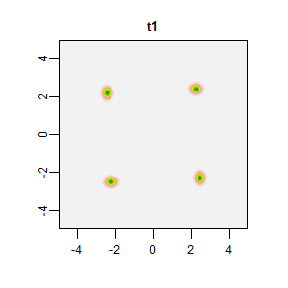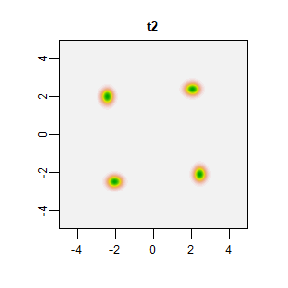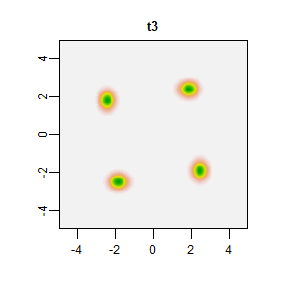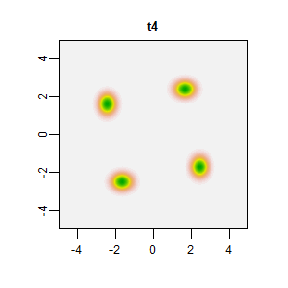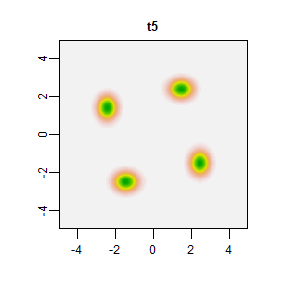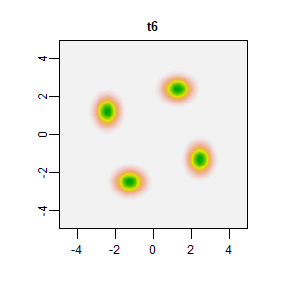To decide which ICvectorfields function to use, the user can consult the table in the overview section of this vignette. We will first use the DispField function estimate displacement based on a pair of images using a grid to define regions of interest from whence displacement will be estimated:

VFdf1 <- DispField(SimStack[], SimStack[], factv1 = 101, facth1 = 101, restricted = TRUE)
VFdf1
#>   rowcent colcent frowmin frowmax fcolmin fcolmax     centx     centy
#> 1      51      51       1     101       1     101 -2.499878  2.499878
#> 2     152      51     102     202       1     101 -2.499878 -2.450861
#> 3      51     152       1     101     102     202  2.450861  2.499878
#> 4     152     152     102     202     102     202  2.450861 -2.450861
#>        dispx      dispy
#> 1  0.0000000 -0.9803443
#> 2  0.9803443  0.0000000
#> 3 -0.9803443  0.0000000
#> 4  0.0000000  0.9803443

The function divides the spatial domain up into sub-grids of 101 by 101 pixels, which in this case, is the size of each of the quadrants of the planar spatial domain. Displacement from the centre of each sub-grid is calculated in the vertical (dispy in the data-frame output printed above) and horizontal (dispx) directions. In the five time steps between t1 and t6, the concentration in the upper left quadrant (first row of table output) moves down by approximately 0.98 spatial units; the concentration in the lower left quadrant (second row of table output) moves to the right by 0.98 spatial units; the concentration in the upper right quadrant (third row of table output) moves to the left by 0.98 spatial units, and the concentration in the lower right quadrant (fourth row of table output) moves up by 0.98 spatial units. An estimate of the speed of moment can be obtained by dividing 0.98 by five time steps, which results in a speed estimate of 0.196 spatial units per unit time, a little under the simulated advection speed of 0.2 spatial units per unit time in all directions. The bias in the estimate is likely due to the diffusion term in the partial differential equation as diffusion obfuscates the impact of advection.

Because speed is constant in the simulation model, the DispFieldST function is appropriate for estimating orthogonal velocity vectors:

VFdf2 <- DispFieldST(SimStack, lag1 = 1, factv1 = 101, facth1 = 101, restricted = TRUE)
VFdf2
#>   rowcent colcent frowmin frowmax fcolmin fcolmax     centx     centy
#> 1      51      51       1     101       1     101 -2.499878  2.499878
#> 2     152      51     102     202       1     101 -2.499878 -2.450861
#> 3      51     152       1     101     102     202  2.450861  2.499878
#> 4     152     152     102     202     102     202  2.450861 -2.450861
#>        dispx      dispy
#> 1  0.0000000 -0.1960689
#> 2  0.1960689  0.0000000
#> 3 -0.1960689  0.0000000
#> 4  0.0000000  0.1960689

The movement speed is estimated as 0.196 units of space per unit time in each of the quadrants and the directions are consistent with simulated advection directions. Note that in the function above, the logical restricted argument is set to TRUE, whereas the default is FALSE. The restricted argument restricts the search for cross-covariance to areas within each of the grids that are designated using the factv1, and facth1 arguments to the function when set to TRUE. When restricted is set to FALSE the algorithm searches the entire spatial domain to look for maximum cross-covariance.

DispFieldST(SimStack, lag1 = 1, factv1 = 101, facth1 = 101, restricted = FALSE)
#>   rowcent colcent frowmin frowmax fcolmin fcolmax     centx     centy
#> 1      51      51       1     101       1     101 -2.499878  2.499878
#> 2     152      51     102     202       1     101 -2.499878 -2.450861
#> 3      51     152       1     101     102     202  2.450861  2.499878
#> 4     152     152     102     202     102     202  2.450861 -2.450861
#>         dispx       dispy
#> 1  0.09803443 -3.87236014
#> 2  3.87236014  0.09803443
#> 3 -3.87236014 -0.09803443
#> 4 -0.09803443  3.87236014

When restricted is set to FALSE as above, the algorithm fails to correctly approximate movement speed because maximum cross-covariance is observed across quadrants. By not restricting the search window, false signals can be interpreted as large movement vectors by the algorithm. It is important, therefore, to critically assess any estimate movement estimated using DIC-based approaches–especially when estimates of speed are unusually large.

To plot vector fields, ICvectorfields depends on on ggplot2 (Wickham 2016) and its extensions in the metR (Campitelli 2021b) and ggnewscale (Campitelli 2021a) packages:

SimVF = ggplot() +
xlim(c(-5, 5)) +
ylim(c(-5, 5)) +
geom_raster(data = SimData,
aes(x = xcoord, y = ycoord, fill = t1)) +
scale_fill_gradient(low = "white", high = "blue", na.value = NA) +
new_scale("fill") +
geom_raster(data = SimData,
aes(x = xcoord, y = ycoord, fill = t6), alpha = 0.5) +
scale_fill_gradient(low = "white", high = "red", na.value = NA) +
geom_vector(data = VFdf2,
aes(x = centx, y = centy,
mag = Mag(dispx, dispy),
angle = Angle(dispx, dispy))) +
theme_bw()
SimVF
#> Warning: Removed 403 rows containing missing values (geom_raster).

#> Warning: Removed 403 rows containing missing values (geom_raster).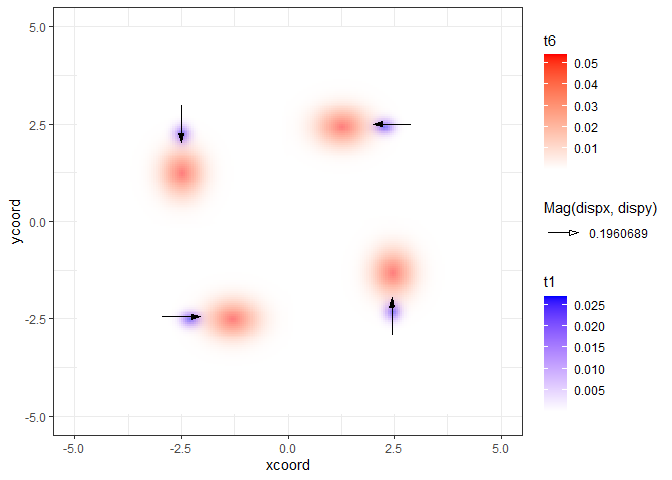## Demonstration using Larch Budmoth Defoliation Data

Aggregated larch budmoth data that were used to demonstrate the existence of wave-trains (Bjørnstad et al. 2002) are provided as part of the ncf package (Bjornstad 2020). In the plot below the first five years of the time series that extends from 1961 to 1998 are plotted.

# import larch budmoth data from ncf package
data(lbm, package = "ncf")

# convert to raster stack
LBMStack <- ICvectorfields::RastStackData(lbm)

# confirming dimension
dim(LBMStack)
#>  19 31 38

# visualizing
terra::plot(LBMStack[], legend = FALSE, main = "1961")
terra::plot(LBMStack[], legend = FALSE, main = "1962")
terra::plot(LBMStack[], legend = FALSE, main = "1963")
terra::plot(LBMStack[], legend = FALSE, main = "1964")
terra::plot(LBMStack[], legend = FALSE, main = "1965")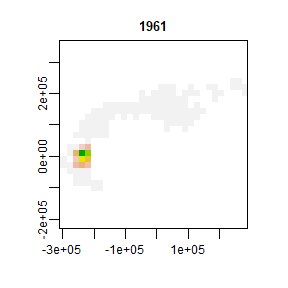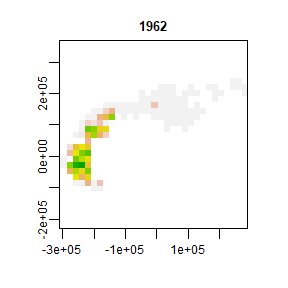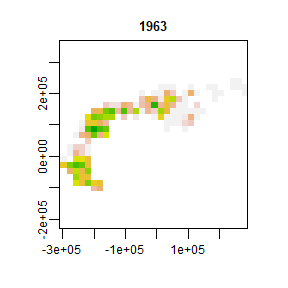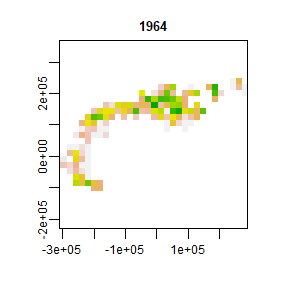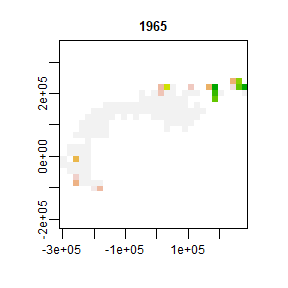Because the geographic extent of the data is large, it is likely that population movement varies from one location to another. For this reason, the DispFieldSTall function is the most appropriate choice to produce vector fields. Previous analyses have also demonstrated that populations appear to move at high speeds of up to two hundred km per year and so the default unrestricted mode (restricted == FALSE) of the function is appropriate. Previous analyses also determined that a lag of between two and three years captured the nonlinearities inherent in the larch budmoth system. Note in the code below, the analysis is restricted to the years 1961 to 1983 (the first 23 layers in the raster stack). The reason for selecting these years is that after 1983, the pattern of spread becomes much less clear and some years show discontinuous jumps in the population when no defoliation was recorded in the middle of an outbreak.

VFdf3 <- DispFieldSTall(LBMStack[[1:23]], lagmax = 3, factv1 = 3, facth1 = 3, restricted = FALSE)

LBMVF1 = ggplot() +
geom_raster(data = lbm,
aes(x = x, y = y,
fill = X1962)) +
scale_fill_gradient(low = "white", high = "blue", na.value = NA) +
new_scale("fill") +
geom_raster(data = lbm,
aes(x = x, y = y, fill = X1964), alpha = 0.5) +
scale_fill_gradient(low = "white", high = "red", na.value = NA) +
geom_vector(data = VFdf3,
aes(x = centx, y = centy,
mag = Mag(dispx, dispy),
angle = Angle(dispx, dispy))) +
theme_bw()
LBMVF1
#> Warning: Raster pixels are placed at uneven horizontal intervals and will be
#> shifted. Consider using geom_tile() instead.

#> Warning: Raster pixels are placed at uneven horizontal intervals and will be
#> shifted. Consider using geom_tile() instead.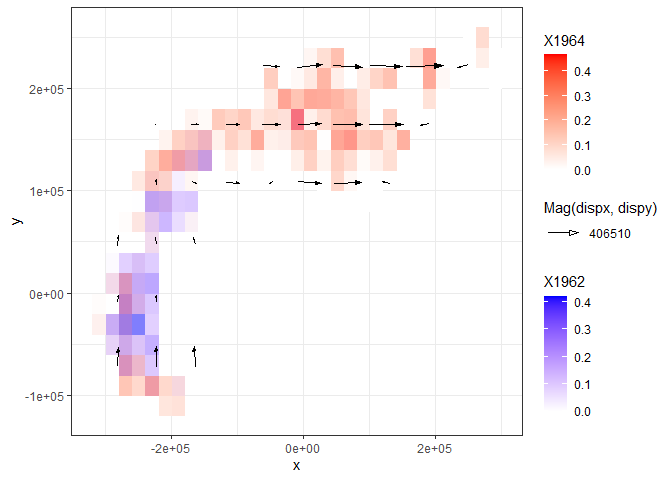The vector field reveals that the true directions of movement are at approximately right angles first to the north and then to the east and not at an angle in between as previously reported. Moreover, the vector field elucidates how directed movement slows down when it changes from northward to eastward spread. Average population movement speed and its standard deviation can be computed in order to construct Wald-type 95 percent confidence intervals on the average population movement speed.

VFdf3$speed <- sqrt((VFdf3$dispx^2) + VFdf3$dispy^2) # subsetting to remove locations where speed is zero VFdf4 <- subset(VFdf3, speed > 0) # computing mean, standard deviation and dimension of data frame # to obtain sample size mean(VFdf4$speed)
#>  175810
sd(VFdf4$speed) #>  110385 dim(VFdf4) #>  29 11 # upper and lower Wald-type 95 percent confidence interval on average speed mean(VFdf4$speed)/1000 + qt(0.975, dim(VFdf4) - 1)*sd(VFdf4$speed)/1000/sqrt(dim(VFdf4) - 1) #>  218.5415 mean(VFdf4$speed)/1000 + qt(0.025, dim(VFdf4) - 1)*sd(VFdf4\$speed)/1000/sqrt(dim(VFdf4) - 1)
#>  133.0786

The estimate of average speed is smaller than previous estimates of east-northeast movement at a speed of 220 km per year (Bjørnstad et al. 2002) and 254 km per year (Johnson, Bjørnstad, and Liebhold 2004). A likely reason for the difference in average speeds between estimates from the literature and estimates produced by ICvectorfields is that both of the referenced estimates project populations onto lines at a variety of angles before estimating speed (Bjornstad 2020), whereas functions in ICvectorfields do not. Therefore, the mostly horizontal and vertical movement vectors evident in the figure produced for larch budmoth above are translated to vectors at an angle using previous methods. Simple geometry shows estimates produced by projecting onto a line are larger than component horizontal and vertical vectors (see R code below):

# the hypotenuse of a right angled triangle whose vertical and horizontal sides are vectors of magnitude
# 176 (coresponding to average speed estimated in the previous R-chunk) has a length of
sqrt((176^2) + (176^2))
#>  248.9016
# (pythagorean theorem)

The hypotenuse of a right angle triangle with velocities in the horizontal and vertical direction of 176 km per year produces a velocity vector in the north-eastern direction with speed equal to 249 km per year–an estimate that is much closer to previous published estimates of larch budmoth population movement speed (Bjørnstad et al. 2002; Johnson, Bjørnstad, and Liebhold 2004).

# Expected Uses of Software

The ICvectorfields software will most likely be useful for exploratory and confirmatory research applications and for visualization of data for hypothesis generation. The caveats that researchers should consider when using ICvectorfields or related approaches are embodied in the often quoted statement that correlation is not causation. The functions in the ICvectorfields package infer displacement based on cross-covariance, which is a form of correlation. Moreover, by changing the arguments of the functions in this package, it is very possible to obtain different results as demonstrated in this vignette. For these reasons, users must be vigilant when using these approaches or any others that rely on cross-correlation or cross-covariance.